Next: Translational motion versus rotational Up: Rotational motion Previous: Torque

## Power and work

Consider a massattached to the end of a light rod of length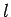whose other end is attached to a fixed pivot. Suppose that the pivot is such that the rod is free to rotate in any direction. Suppose, further, that a force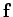is applied to the mass, whose instantaneous angular velocity about an axis of rotation passing through the pivot is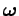.

Letbe the instantaneous velocity of the mass. We know that the rate at which the forceperforms work on the mass--otherwise known as the power--is given by (see Sect. 5.8)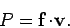(369)

However, we also know that (see Sect. 8.4)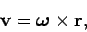(370)

where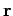is the vector displacement of the mass from the pivot. Hence, we can write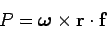(371)

(note that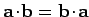).

Now, for any three vectors,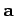,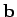, and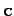, we can write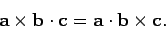(372)

This theorem is easily proved by expanding the vector and scalar products in component form using the definitions (319) and (321). It follows that Eq. (371) can be rewritten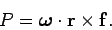(373)

However,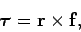(374)

where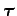is the torque associated with forceabout an axis of rotation passing through the pivot. Hence, we obtain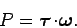(375)

In other words, the rate at which a torque performs work on the object upon which it acts is the scalar product of the torque and the angular velocity of the object. Note the great similarity between Eq. (369) and Eq. (375).

Now the relationship between work,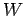, and power,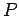, is simply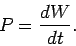(376)

Likewise, the relationship between angular velocity,, and angle of rotation,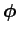, is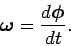(377)

It follows that Eq. (375) can be rewritten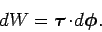(378)

Integration yields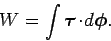(379)

Note that this is a good definition, since it only involves an infinitesimal rotation vector,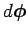. Recall, from Sect. 8.3, that it is impossible to define a finite rotation vector. For the case of translational motion, the analogous expression to the above is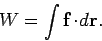(380)

Here,is the force, and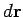is an element of displacement of the body upon which the force acts.

Although Eqs. (375) and (379) were derived for the special case of the rotation of a mass attached to the end of a light rod, they are, nevertheless, completely general.

Consider, finally, the special case in which the torque is aligned with the angular velocity, and both are constant in time. In this case, the rate at which the torque performs work is simply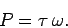(381)

Likewise, the net work performed by the torque in twisting the body upon which it acts through an angle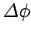is just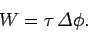(382)Next: Translational motion versus rotational Up: Rotational motion Previous: Torque
Richard Fitzpatrick 2006-02-02